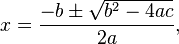# Question about freely falling objects

## Homework Statement

Q: A stone is thrown vertically upwards with initial speed of 5 m/s from edge of a cliff 30 m high. Find the time needed for the stone to reach the ground (in seconds).

## Homework Equations

1. (h= Vo t - 0.5 g t^2)## The Attempt at a Solution

First I will use this equation: (h= Vo t - 0.5 g t^2)
-30 = (5) t - 5 t^2
Now I divide by 5 and it will be like this
t^2 - t - 6
Then, I use the quadratic equation
$\frac{-(-1)+\sqrt{(-1)^2-4(1)(-6)}}{2(1)}$

The answer is 3, is that right?

Another question, I am not sure..
maybe I need to calculate the upward vertical distance it will go first.. then I sum it with the actual height which is 30 m?

The answer is 3, is that right?
Looks right to me.

simple calculation..
considering down as positive...
h=ut+1/2at.t
h=30 m
a= 10 m/s.s
u=-5m/s

30=-5t+5t.t
t=3 s

simple calculation..
considering down as positive...
h=ut+1/2at.t
h=30 m
a= 10 m/s.s
u=-5m/s

30=-5t+5t.t
t=3 s